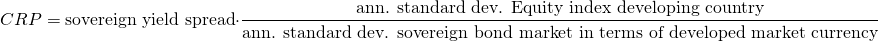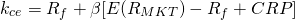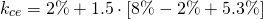A country risk premium (CRP) is used to estimate the cost of capital for developing countries. For these countries, the equity beta estimated using the Capital Asset Pricing Model (CAPM) does not necessarily capture country risk. To measure the increased risk, analysts add a CRP to the market risk premium used in the CAPM.

On this page, we provide the country risk premium formula, illustrate how to adjust the CAPM formula to include the CRP, and provide a country risk premium example.

To estimate the risk of the developing country, we make use of its sovereign yield spread. This is the difference in yields between the developing country and the developed country, both expressed in the developed country’s currency and with a similar maturity. Next, we adjust the sovereign yield spread by the ratio of the volatility of the developing country’s equity market and its government bond market. This is country risk premium.

Expressing the above definition using a formula, the CRP formula equalswhere the sovereign yield spread is equal to the difference between the yield on the government bond in the developing country and the government bond yield of a bond from the investor’s home country. As we mentioned in the section on the definition, we clearly see that the CRP formula scales the sovereign yield spread using the ratio of the volatilities.

Next, we need to include the above CRP in the CAPM formula. To do this, we increase the required rate of return as followsNext, let’s consider an example that shows how to calculate the country risk premium.

Suppose we want to estimate cost of equity for a country for which we calculated the CRP. We have the following information:

• Project beta = 1.5
• Risk-free rate = 2%
• Expected market return = 8%
• Country risk premium = 5.3%

Then the cost of equity equalsor 18.9%. How we calculated the 5.3% premium using the formula we discussed above is explained in the Excel spreadsheet below.

## Summary

The CRP is a way to adjust a project’s beta such that the required rate of return reflects country risk. This is especially important in the case of developing countries.

### CRP calculator

Want to have an implementation of CAPM that incorporate the CRP? Download the Excel file here: Country Risk Premium